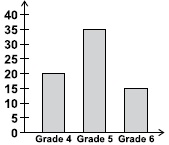# User Forum

Subject :IMO    Class : Class 4

At a school, fourth, fifth and sixth graders sold flowers as a fundraiser. The adjoining graph shows how many flowers were sold by each grade. How many flowers did the students sell in all?A20
B35
C40
D70

Class : Class 1

(D)

Number of flowers sold by students of grade 4 = 20

Number of flowers sold by students of grade 5 = 35

Number of flowers sold by students of grade 6 = 15

Total number of flowers sold by all students = 20+35+15 =70